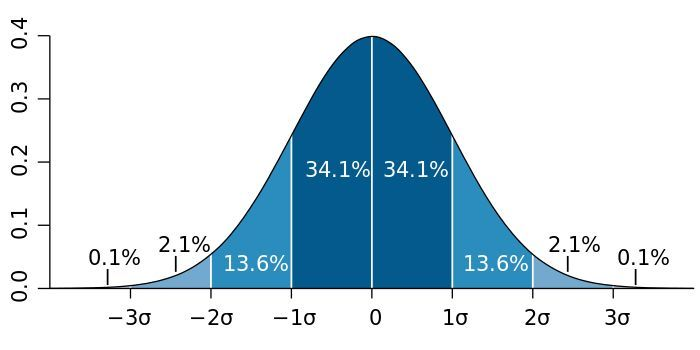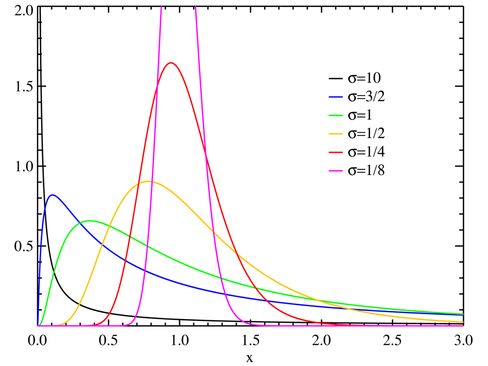## 正态分布的一些应用

$$\sigma_\mu=\frac{\sigma}{\sqrt{N}}$$ $$\sigma_\Sigma= \sigma \sqrt{N}$$## 对数正态分布